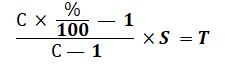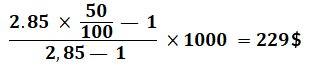Betting school

# Kelly’s criterion in sports bettingThe purpose of the betting strategy "Kelly's Criterion" is to determine the size of each upcoming bet for more reasonable management of your game account. It is believed that this method helps to protect the player from losing the entirety of their funds, as it does not provide for a fixed bet size: the player independently assesses the probability of the event on which he plans to bet, and, based on this, calculates the optimal bid size under a special formula.

## When to use Kelly's criterion in betting?

Kelly's criterion is more suited for those who already have some experience of making bets with bookmakers, as the success of this strategy is determined, most of all, by the player’s ability to independently determine the probability of an event.

In fact, the strategy can be considered as a kind of competition between the player and the bookmaker, where the winner is the one who was able to more correctly determine the probability of the event.

Kelly's criterion is considered to be an improved copy of Value Betting, and it is worth using in cases where the bookmaker underestimated the probability of an event and, accordingly, offered an inflated factor. Among the clients of bookmakers, this event is called- "value."

The disadvantages of Kelly's criterion include the probability of an incorrect self-assessment of the event by the player. So, if a player overestimated the probability of an event, he will lose. If he underestimated it, then it will lead to a smaller winning spree.

## How to play by Kelly's criterion: calculation formula and examples

The Kelly criterion formula gives you the ability to calculate what the optimal bet size should be for a particular event. Again, this size will only be optimal if the bookmaker underestimated the probability of the event, and the player guessed it correctly.

To translate the coefficient into a percentage probability, you can use a simple formula: take 100% divided by the coefficient.

For example, let's say Barcelona face Sevilla and the odds of Barca winning are 1.40. To understand what the probability of a Barca victory is in a percentage, at least what the bookmaker believes is correct, we predict according to the above formula: 100/1.40 = 71.4%. Thus, the bookmaker believes that Barcelona will win with a probability of 71%.

The player making the bet believes that the probability of success of Barca is 60%. Therefore, the proposed quote is not overvalued, because the probability of the outcome, according to the player, is lower than what is suggested by the bookmaker. That means it is not a bet for Kelly's test.

In order to correctly calculate the optimal size of the rate according to the Kelly criterion, we use the following formula:C - odds offered by the bookmaker

% is the percentage of probability of a player's assessment event

S - bankroll size

T is the required bet size

### Let's look at an example

Manchester United host Tottenham at Old Trafford. For the probability of success for the "Red Devils" the bookmaker gives a factor of 2.85, therefore, is estimating the possible victory of MU at 35%. The bettor, on the other hand, assumes that the probability of this event is 50% (value). It is still necessary to calculate the amount of bet that should be made on this event using the above formula. Let's say the size of our bankroll is 1000 U.S.

The original data for the formula:

2.85 - odds offered by the bookmaker

50 is the percentage of probability a player believes

1000 - bank size

T is the amount of bet that needs to be calculated.Based on the calculations, we see that here we need to put 22.9% of the total size of the bankroll.

Summing up all of the above, again we remind you that this strategy of betting is more suitable for experienced players, as it is always difficult to determine the probability of an event without the help of the odds line.

Post by: